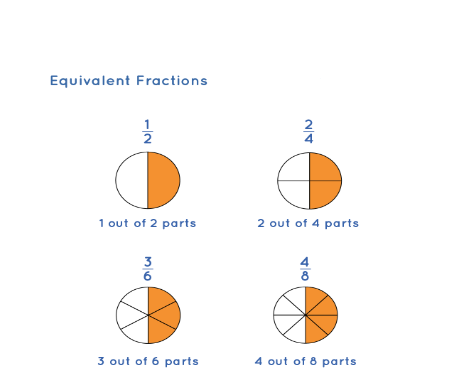To find fractions equivalent to a given fraction​

## Objective

To find fractions equivalent to a given fraction​

### Fraction

• A fraction is a number representing part of a whole.
• The whole may be a single object or a group of objects.
• A number expressed as a quotient, in which a numerator is divided by a denominator called fraction.

### Equivalent fractions

• It is defined as those fractions which are equal to the same value irrespective of their numerators and denominators.
• For example, both 6/12 and 4/8 are equal to 1/2, when simplified, which means they are equivalent in nature.

### Equivalent Fractions Examples

1/2, 2/4, 3/6, 4/8 are equivalent fractions. Let us see how their values are equal. We will represent each of these fractions as circles with shaded parts. It can be seen that the shaded parts in all the figures represent the same portion if seen as a whole.Fig.(1) Fraction

Here, we can see that the amount of shaded portion is the same in all the circles. Hence, 1/2, 2/4, 3/6, and 4/8 are equivalent fractions.